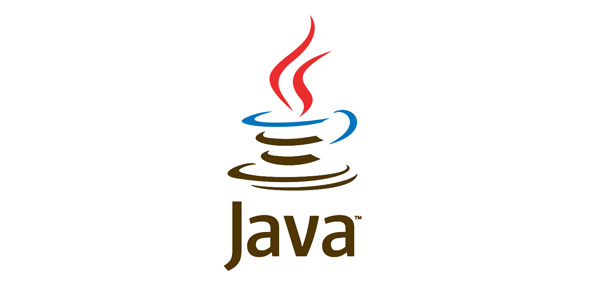# Online Test On OOP Using Java

71 Questions | Attempts: 321SettingsNo Negative marking Attempt questions as many you can

• 1.
Following code will result in: int a = 3.5;
• A.

Compilation error

• B.

Runtime error

• 2.
Following code will result in: int a1 = 5; double a2 = (float)a1;
• A.

Runtime error

• B.

No errors

• 3.
Following code will result in: int a = 9/0;
• A.

Compilation error: Divisions must be in a try block.

• B.

Runtime Exception

• 4.
Following code will result in: float a = 9/0
• A.

Compilation error: DivideByZeroException

• B.

Runtime Exception

• 5.
A class can be transient
• A.

True

• B.

False

• 6.
Following code will result in: class A { int b = 1; public static void main(String [] args) { System.out.println("b is " + b); }}
• A.

Compilation error

• B.

Runtime Error

• 7.
Following code will result in: class A { public static void main(String [] args) {A a = new B(); }} class B extends A {}
• A.

Compile error

• B.

No error

• 8.
Following code will result in: class A { public static void main(String [] args) {A a = new B(); }} class B extends A {}
• A.

Runtime Exception

• B.

No errors

• 9.
Methods that are marked protected can be called in any subclass of that class.
• A.

True

• B.

False

• 10.
An abstract class can have non-abstract methods
• A.

True

• B.

False

• 11.
Java keywords are written in lowercase as well as uppercase
• A.

True

• B.

False

• 12.
What is an instanceof
• A.

A methods in object

• B.

An operator and keyword

• 13.
Primitive datatypes are allocated on a stack
• A.

True

• B.

False

• 14.
Can you compare a boolean to an integer?
• A.

Yes

• B.

No

• 15.
If class A implements an interface does it need to implement all methods of that interface?
• A.

Yes, always

• B.

No, not when A is abstract

• 16.
Integer a = new Integer(2); Integer b = new Integer(2); What happens when you do if (a==b)?
• A.

True

• B.

FALSE

• 17.
The methods wait(), notify() and notifyAll() in Object need to be called from synchronized pieces of code.
• A.

True

• B.

False

• 18.
Inner classes can be defined within methods
• A.

True

• B.

False

• 19.
Synchronized is a keyword to tell a Thread to grab an Object lock before continuing execution.
• A.

True

• B.

False

• 20.
The default statement of a switch is always executed
• A.

True

• B.

False

• 21.
How can you prevent a member variable from becoming serialized?
• A.

By marking it volatile

• B.

By marking it transient

• 22.
What is Java (in regard to Computer Science) ?
• A.

A type of coffee

• B.

AN OBJECT-ORIENTED PROGRAMMING LANGUAGE

• 23.
WHAT IS AN APPLET?
• A.

A JAVA PROGRAM THAT IS RUN THROUGH A WEB BROWSER

• B.

A type of coffee

• 24.
Java runs on _______.
• A.

Windows

• B.

All of the Above

• C.

Unix/Linux

• 25.
Why can't the whole program just consist of the one line that does the painting ?
• A.

In Java, to create an applet, you can't call on functions without defining a class to call them.

• B.

The drawString function is not defined without the "import" statements at the top.

• C.

All of the above.

## Related TopicsBack to top
×

Wait!
Here's an interesting quiz for you.